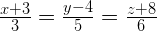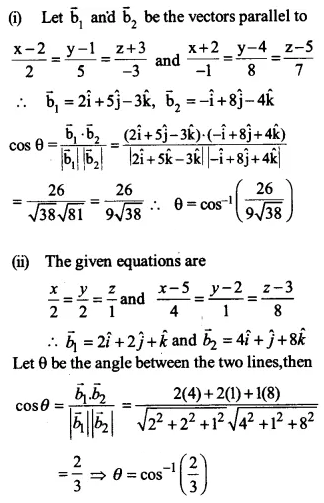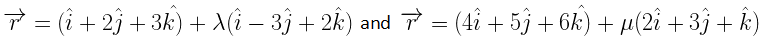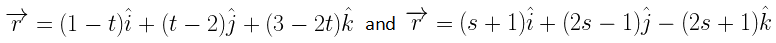NCERT Solutions for Maths Class 12 Exercise 11.2

# NCERT Solutions for Maths Class 12 Exercise 11.2

## NCERT Solutions for Maths Class 12 Exercise 11.2

Maths Class 12 Ex 11.2 Question 1.

Show that the three lines with direction cosines (12/13, 3/13, 4/13); (4/13, 12/13, 3/13); (3/13, 4/13, 12/13); are mutually perpendicular.

Solution:

Let the lines be L1, L2 and L3.
For line L1, direction cosines are: l1 = 12/13; m1 = –3/13; n1 = –4/13;
For line L2, direction cosines are: l2 =
4/13; m2 = 12/13; n2 = 3/13;
For line L3, direction cosines are: l3 =
3/13; m3 = –4/13; n3 = 12/13;
Let the angle between lines L1 and L2 is θ1.

Then, cos θ1 = l1 l2 + m1 m2 + n1 n2

= (12/13 × 4/13) + (3/13 × 12/13) + (4/13 × 3/13)

= 48/169 – 36/169 – 12/169 = 0

θ1 = 90˚

Thus, the angle between lines L1 and L2 is 90˚.

Let the angle between lines L2 and L3 is θ2.

Then, cos θ2 = l2 l3 + m2 m3 + n2 n3

= (4/13 × 3/13) + (12/13 × 4/13) + (3/13 × 12/13)

= 12/169 – 48/169 + 36/169 = 0

θ2 = 90˚

Thus, the angle between lines L2 and L3 is 90˚.

Let the angle between lines L1 and L3 is θ3.

Then, cos θ3 = l1 l3 + m1 m3 + n1 n3

= (12/13 × 3/13) + (3/13 × 4/13) + (4/13 × 12/13)

= 36/169 + 12/169 – 48/169 = 0

θ3 = 90˚

Thus, the angle between lines L1 and L3 is 90˚.

Hence, the given lines are mutually perpendicular.

Maths Class 12 Ex 11.2 Question 2.

Show that the line through the points (1, –1, 2), (3, 4, –2) is perpendicular to the line through the points (0, 3, 2) and (3, 5, 6).

Solution:

Let A and B be the points (1, –1, 2) and (3, 4, –2), respectively.

Direction ratios of AB are: a1 = 3 – 1 = 2, b1 = 4 – (–1) = 5, c1 = –2 – 2 = –4

Let C and D be the points (0, 3, 2) and (3, 5, 6), respectively.

Direction ratios of CD are: a2 = 3 – 0 = 3, b2 = 5 – 3 = 2, c2 = 6 – 2 = 4

Now, AB is perpendicular to CD if

a1a2 + b1b2 + c1c2 = 0

2 × 3 + 5 × 2 + (–4) × 4 = 6 + 10 – 16 = 0

Hence, the lines AB and CD are perpendicular.

Maths Class 12 Ex 11.2 Question 3.

Show that the line through the points (4, 7, 8), (2, 3, 4) is parallel to the line through the points (–1, –2, 1) and (1, 2, 5).

Solution:

Let the points be A(4, 7, 8), B(2, 3, 4), C(–1, –2, 1) and D(1, 2, 5).
Now, direction ratios of AB are:

Maths Class 12 Ex 11.2 Question 4.

Find the equation of the line which passes through the point (1, 2, 3) and is parallel to the vector 3i + 2j – 2k.

Solution:

Equation of the line passing through the point

Maths Class 12 Ex 11.2 Question 5.

Find the equation of the line in vector and in Cartesian form that passes through the point with position vector 2ij + 4k and is in the direction i + 2jk.

Solution:

The vector equation of a line passing through a point with position vector a = 2ij + 4k and parallel to the vector b = i + 2jk is

Maths Class 12 Ex 11.2 Question 6.

Find the Cartesian equation of the line which passes through the point P(–2, 4, –5) and parallel to the line is given by.

Solution:

Given that the line passes through the point (–2, 4, –5) and parallel to the line given by.

Thus, the line passing through P(x1, y1, z1) where x1 = –2, y1 = 4, z1 = –5 and the direction ratios a1 = 3, b1 = 5, c1 = 6 is

Maths Class 12 Ex 11.2 Question 7.

Solution:

Clearly (i) passes through the point (5, –4, 6) and has 3, 7, 2 as its direction ratios.
Line (i) passes through the point A with

Maths Class 12 Ex 11.2 Question 8.

Find the vector and the Cartesian equations of the lines that passes through the origin and (5, –2, 3).

Solution:

Given that the line passes through point A (0, 0, 0) and B(5, –2, 3).
Therefore, a = 0i + 0j + 0k and b = 5i – 2j + 3k
Direction ratios of the line passing through the

Maths Class 12 Ex 11.2 Question 9.

Find the vector and Cartesian equations of the line that passes through the points (3, –2, –5), (3, –2, 6).

Solution:

The PQ passes through the point P(3, –2, –5).

Maths Class 12 Ex 11.2 Question 10.

Solution:

(i) Let θ be the angle between the given lines.
The given lines are parallel to the vectors

Maths Class 12 Ex 11.2 Question 11.

Solution:Maths Class 12 Ex 11.2 Question 12.

Solution:

The given equations are not in the standard form.
The equations of given lines in standard form are

Maths Class 12 Ex 11.2 Question 13.

Solution:

Then the direction ratios of the given lines are: a1 = 7, b1 = –5, c1 = 1 and a2 = 1, b2 = 2, c2 = 3

Maths Class 12 Ex 11.2 Question 14.

Solution:

Maths Class 12 Ex 11.2 Question 15.

Solution:

Shortest distance between the lines

Maths Class 12 Ex 11.2 Question 16.

Find the shortest distance between the lines whose vector equations are:Solution:

Maths Class 12 Ex 11.2 Question 17.

Find the shortest distance between the lines whose vector equations areSolution: